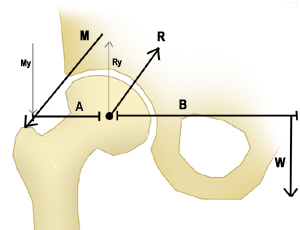358ms
Updated: 1/16/2022

# Hip Biomechanics

0%
Topic
Review Topic
0
0
0%
0%
Flashcards
3
N/A
N/A
Questions
5
0
0
0%
0%
Evidence
10
0
0
0%
0%
Videos / Pods
1
0%
0%
Cases
1
Topic
Images• Joint Biomechanics Definitions
• Joint reaction force defined as force generated within a joint in response to forces acting on the joint
• in the hip, it is the result of the need to balance the moment arms of the body weight and abductor tension
• maintains a level pelvis
• Coupled forces
• when two movements and associated forces are coupled
• Joint congruence
• relates to fit of two articular surfaces
• high congruence increases joint contact area
• Instant center of rotation
• point about which a joint rotates
• often changes during rotation due to joint translation
• center of gravity of human is just anterior to S2
• Friction and lubrication
• not a function of contact area
• lubrication decreases friction
• examples
• coefficient of friction of human joints is .002 to .04
• TJA (metal on PE) is .05 to .15
• Free Body Analysis Definitions
• Newtons laws
• first law
• if there is no net force on an object, its velocity remains constant
• second law
• force equals mass multiplied by acceleration
• F=ma
• third law
• when a first body exerts a force on a second body, the second body exerts a force that is equal in magnitude and opposite in direction on the first body
• F2=-F1
• Force
• definition
• a push or pull on an object resulting from the object's interaction with another object
• equation
• force = mass x acceleration, F=ma
• 1 Newton = force required to give 1 kg mass an acceleration of 1 m/s2
• Vector
• definition
• a quantity that contains both direction and magnitude
• scalar quantities do not have direction
• forces and velocity can be broken down into vectors
• Moment (torque)
• definition
• the tendency of a force to rotate a body around an axis
• equation
• moment (torque) = force(perpendicular) X distance
• Work
• definition
• when a force acts upon an object to create displacement
• equation
• work = force (vector parallel to displacement) x distance
• Energy
• definition
• ability of an object to perform work
• classification
• potential energy
• equation
• U (potential energy) = mass x gravity x height
• kinetic energy
• equation
• KE (kinetic energy) = 1/2mv2
• Hip Free Body Analysis
• Free body analysis diagram• W = gravitational force
• weight of the body minus weight of ipsilateral extremity (or 5/6 body weight)
• M = abductor muscle force
• R = joint reaction force
• can reach 3 to 6 times body weight
• Solving for joint reaction force (R)
• step 1: calculate My
• principle
• sum of all moments equals 0
• in this case, the moments are created by My and W
• equation
• (A x My) + (B x W) = 0
• assume A = 5cm and B = 12.5cm (this information will be given to you)
• My = 2.5W
• step 2: calculate Ry
• Ry = My + W
• Ry = 2.5W + W
• Ry = 3.5W
• step 3: calculate R
• R = Ry / (cos 30°)
• R = 3.5W / (cos 30°)
• R = ~4W
• Clinical Implications
• Actions that decrease joint reaction force include
• increase in ratio of A/B (shift center of rotation medially)
• acetabular side
• moving acetabular component medial, inferior, and anterior
• femoral side
• increasing offset of femoral component
• long stem prosthesis
• lateralization of greater trochanter
• by using increased offset neck/prosthesis
• varus neck-shaft angulation
• increases shear across joint
• patient's gait
• shifting body weight over affected hip
• this results in Trendelenburg gait
• cane in contralateral hand
• reduces abductor muscle pull and decreases the moment arm between the center of gravity and the femoral head
• carrying load in ipsilateral hand
• produces additional downward moment on same side of rotational point
• Actions that increase joint reaction force include
• valgus neck-shaft angulation
• decreases shear across joint
Flashcards (3)
Cards
1 of 3
Questions (5)

(OBQ11.221) A cane held in the contralateral hand reduces joint reactive forces through the affected hip approximately 50% by which of the following mechanisms?

QID: 3644
1

Reducing hip abductor muscle pull

73%

(3543/4871)

2

Increasing hip flexor muscle pull

1%

(42/4871)

3

Moving the center of rotation for the femoroacetabular joint

21%

(1023/4871)

4

Increasing joint congruence at the femoroacetabular joint

1%

(54/4871)

5

Moving the center of gravity posterior to the second sacral vertebra

3%

(170/4871)

L 3 C

Select Answer to see Preferred ResponseSorry, this question is for

(OBQ09.172) Figure A represents a free body diagram of the hip of a patient standing on the right leg. The forces and distances are labeled on the diagram and the resulting hip joint force (J) = 1800N. What is the resultant value for J when the acetabular component is medialized given the new distances shown in Figure B?

QID: 2985
FIGURES:
1

1000N

5%

(178/3260)

2

1200N

64%

(2094/3260)

3

1800N

8%

(250/3260)

4

2200N

10%

(326/3260)

5

3600N

11%

(356/3260)

L 3 C

Select Answer to see Preferred ResponseSorry, this question is for

(OBQ05.76) Patients display a Trendelenburg gait to compensate for weakness in which of the following muscle groups?

QID: 962
1

1%

(22/1633)

2

hip abductors

97%

(1576/1633)

3

hip flexors

1%

(11/1633)

4

knee extensors

0%

(2/1633)

5

hip extensors

0%

(8/1633)

L 1 D

Select Answer to see Preferred Response

Evidence (10)
VIDEOS & PODCASTS (2)
CASES (1)